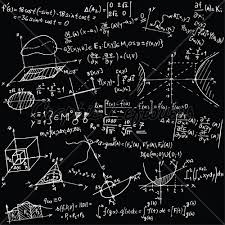# Equation 20

In given equation: 8/9-4/5=2/9+x, find x

x =  -0.1333

### Step-by-step explanation:

8/9-4/5=2/9+x

45x = -6

x = -2/15 ≈ -0.133333

Our simple equation calculator calculates it.Did you find an error or inaccuracy? Feel free to write us. Thank you!Tips to related online calculators
Need help to calculate sum, simplify or multiply fractions? Try our fraction calculator.
Do you have a linear equation or system of equations and looking for its solution? Or do you have a quadratic equation?

## Related math problems and questions:

• Find the 24Find the difference between 2/7 and 1/21
• FractionsSort fractions z1 = (6)/(11); z2 = (10)/(21); z3 = (19)/(22) by its size. Result write as three serial numbers 1,2,3.
• Simple equation 324 = n • 27, solve for n
• Equation 25Solve following simple equation: 3/4(x+5)=1/2(x+9)
• RegroupingSubtract mixed number with regrouping: 11 17/20- 6 19/20
• Find the meanFind the number between 13 and 29.
• Fractions and mixed numerals(a) Convert the following mixed numbers to improper fractions. i. 3 5/8 ii. 7 7/6 (b) Convert the following improper fraction to mixed number. i. 13/4 ii. 78/5 (c) Simplify these fractions to their lowest terms. i. 36/42 ii. 27/45 2. evaluate following ex
• 6/24 change6/24 change to a fraction in lowest terms
• EquationFind x: x + 1/2 = 1/3
• Find the 28Find the area of a square with side length 4/5 yard.
• Seven timesWhich number seven times is just as higher as 27, how much is smaller than 29?
• Find unknownFind unknown numerator: 4/8 + _/8 = 1
• Unknown number 6Determine x if 1/6 of x is equal to 2/5 of the number 24.
• Find unknown 2Find unknown denominator: 2/3 -5/? = 1/4
• Evaluate - lowest termsEvaluate: 16/25 - 11/25 (Express answer as a fraction reduced to lowest terms. )
• Simple equation 2Find X in this simple equation: X/9 = 96/108
• DayWhat part of the day are 23 hours 22 minutes? Express as a decimal number.